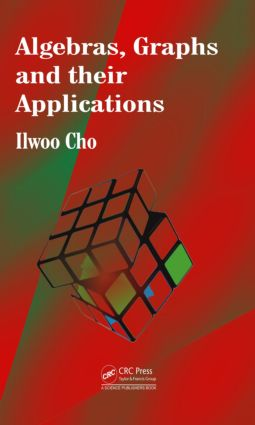Algebras, Graphs and their Applications

1st Edition

CRC Press

444 pages

Hardback: 9781466590199
pub: 2013-09-11
\$135.00
x
eBook (VitalSource) : 9780429073885
pub: 2013-09-11
from \$28.98

FREE Standard Shipping!

Description

This book introduces the study of algebra induced by combinatorial objects called directed graphs. These graphs are used as tools in the analysis of graph-theoretic problems and in the characterization and solution of analytic problems. The book presents recent research in operator algebra theory connected with discrete and combinatorial mathematical objects. It also covers tools and methods from a variety of mathematical areas, including algebra, operator theory, and combinatorics, and offers numerous applications of fractal theory, entropy theory, K-theory, and index theory.

Algebra on Graphs

Introduction

Preliminaries

Groupoids under Operations

Operations on Graphs

Representations and Operator Algebras of Graph Groupoids

Introduction

Partial Isometries

Graph von Neumann Algebras

M-Diagonal Graph W*-Probability Spaces

C*-Subalgebras Generated by Partial Isometries

Operator Theory on Graphs

Introduction

Normality of Graph Operators

Operators in Free Group Factors

Graph Operators Induced by Regular Trees

Fractal on Graph Groupoids

Introduction

Labeled Graph Groupoids and Graph Automata

Graph Fractaloids

Labeling Operators of Graph Fractaloids

Graph-Theoretical Characterization

Fractal Graphs Constructed by Fractal Graphs

Fractal Pairs of Graph Fractaloids

Equivalence Classes of Graph Fractaloids

Completely Finite Fractalization

Fractalized-Graph von Neumann Algebras

Fractalized Labeling Operators

Entropy Theory on Graphs

Entropy

Entropy on Finite Graphs

Entropy of Finite Fractal Graphs

Jones Index Theory on Graph Groupoids

Introduction

Quotient Graphs and Graph Index

Watatani's Extended Jones Index Theory

Index Theory on Graph von Neumann Algebras

Basic Construction Induced by Full-Vertex Subgraph Inclusion

Ladders and Nets on Graph von Neumann Algebras

Index-Morphisms

Network Theory on Graphs

Electric Resistance Network Theory

Representations of ERNs

ERN-Actions on Energy Hilbert Spaces

Free Structures Induced by ERN-Groupoids

K-Theory on Graphs

Introduction

K-Theory

Projections in AG

Projections in M∞ю(AG)

K0-Groups of AG